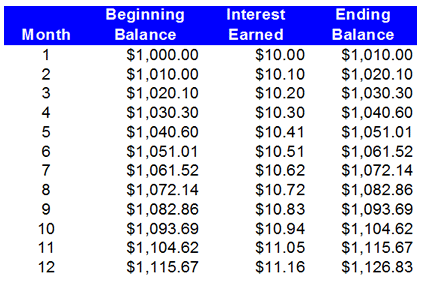# Annual Equivalent Rate (AER)

Updated July 4, 2021

## What is the Annual Equivalent Rate (AER)?

Same as the effective annual interest rate, the annual equivalent (AER) rate is the rate of interest an investor earns in a year after accounting for the effects of compounding. The formula for AER is:

(1 + i/n)n - 1

Where:

i = the stated annual interest rate

n = the number of compounding periods in one year

## How Does the Annual Equivalent Rate (AER) Work?

For example, let’s assume you buy a certificate deposit with a 12% stated annual interest rate. If the bank compounds the interest every month (that is, 12 times per year), then using this information and the formula above, the AER on the CD is:

(1 + .12/12)12 - 1 = .12683 or 12.683%

Let’s look at it from another angle. Assume you put \$1,000 into the 12% CD. Over 12 months, the investment will look like this:The percentage change from \$1,000 to \$1,126.83 is (\$1,126.83 - \$1,000)/\$1,000 = .12683 or 12.683%. Even though the bank has advertised a 12% interest rate, your money actually grew by 12.683%.

## Why Does the Annual Equivalent Rate (AER) Matter?

The AER rate takes compounding into consideration and is thus almost always higher than the stated annual interest rate. It is a useful tool for evaluating the true return on an investment or the true interest rate paid on a loan, though it often does not include one-time charges ("front-end fees").

All of our content is verified for accuracy by Paul Tracy and our team of certified financial experts. We pride ourselves on quality, research, and transparency, and we value your feedback. Below you'll find answers to some of the most common reader questions about Annual Equivalent Rate (AER).
##### Be the first to ask a question

Paul Tracy - 140Paul has been a respected figure in the financial markets for more than two decades. Prior to starting InvestingAnswers, Paul founded and managed one of the most influential investment research firms in America, with more than 3 million monthly readers.